# 累积剩余熵的若干基本性质及其在股票分析中的应用The Basic Properties of Accumulated Residual Entropy and Its Application in Stock Analysis

DOI: 10.12677/PM.2018.81013, PDF, HTML, XML, 下载: 970  浏览: 2,880  科研立项经费支持

Abstract: On the basis of information entropy, we further study some properties of the cumulative residual entropy, and the cumulative residual entropy model applied in risk measure. In practical applica-tions, the selection of eight stocks, collect the daily closing price data, using SPSS software to draw the cumulative residual entropy and the standard deviation of the diagram. It is concluded that the cumulative residual entropy and the standard deviation of the linear relationship, and because of the cumulative residual entropy of a broader scope than variance, namely in the risk measurement, the cumulative residual entropy model has more advantages than the variance.

1. 引言

20世纪，shannon提出了一个对离散随机变量不确定性的度量，即shannon熵，这与风险的本质相吻合。近年来，越来越引起人们的关注与研究，并将熵应用于金融投资组合、风险度量和数据压缩等领域中。本文中如无特别说明，总假定log是以2为底的对数。对于离散随机变量 $X$ ，其shannon熵  的定

$\left\{1,2,3,4,5,6,7,8\right\}$ ，每一个的取值概率都为 $\frac{1}{8}$$Y$ 的取值空间是 $\left\{1,2,3,4,5,6,7,10000\right\}$ ，每一个的取值概率也都为 $\frac{1}{8}$ ，则 $H\left(X\right)=-\underset{i=1}{\overset{8}{\sum }}\frac{1}{8}\mathrm{log}\frac{1}{8}=\frac{27}{8}=H\left(Y\right)$ ，即 $X$$Y$ 的shannon熵是一样的，但如果 $X$$Y$ 代表的

2. 累积剩余熵的基本性质

$\epsilon \left(X\right)=-{\int }_{{R}_{+}^{N}}P\left(|X|>\lambda \right)\mathrm{log}P\left(|X|>\lambda \right)\text{d}\lambda$

$|{X}_{i}|>{\lambda }_{i},i=1,\cdots ,N,{R}_{+}^{N}=\left\{{x}_{i}\in {R}^{N};{x}_{i}\ge 0\right\}$ .

${X}_{1},{X}_{2},\cdots ,{X}_{n}$ 是正的且是独立同分布的随机变量， $F$ 是其分布函数，令 ${F}_{n}$$n$ 个样本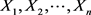的经验分布，且每个样本的概率都是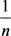，令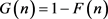，则经验分布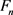的CRE为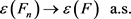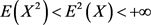,

Pareto分布是一种比较常见的厚尾分布，厚尾分布是金融市场中比较常见的分布。首先，我们了解一下Pareto分布的密度函数，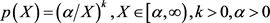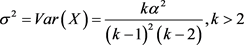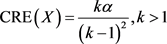1) 均匀分布的CRE。，则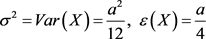,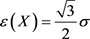2) 指数分布的CRE。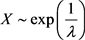，则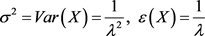,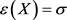3) Pareto分布的CRE。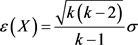3. 实证分析Table 1. The amount and probability of A winning the turntable ATable 2. The amount of money and probability of winning the turntable B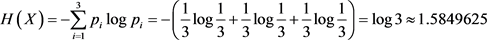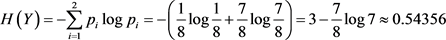,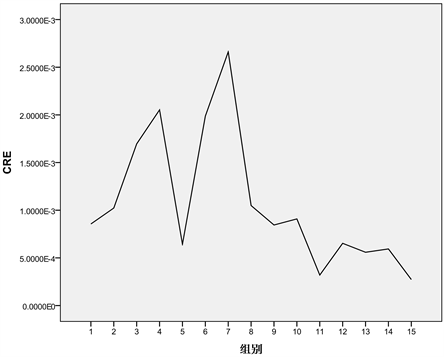Figure 1. Sequence diagram of CRE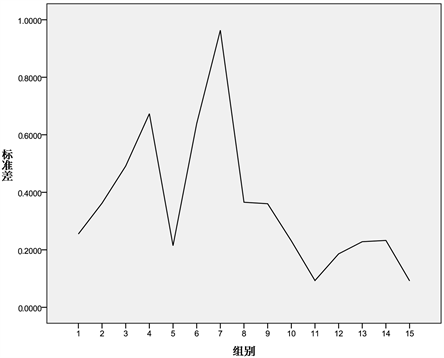Figure 2. The standard deviation sequence diagramFigure 3. The linear correlation between standard deviation and CRE

  Mar kowitz, H. (1952) Portfolio selection. Journal of Finance, 7, 71-91.  Jorion, P. (1996) Measure the Risk in Value at Risk. Financial Analysts Journal, 11, 47-55.  Sharpe, W.F. (1964) Capital Asset Prices: A Theory of Market Equilibrium under Con-ditions of Risk. Journal of Finance, 19, 425-442.  Wirch, J.I. and Hardy, M.R. (1999) A Synthesis of Risk Measures for Capital Adequacy. Insurance: Mathematics and Economics, 25, 337-347.  杨丽娟, 李兴斯. 度量尾部风险的剩余熵模型[J]. 运筹与管理, 2010, 19(6): 88-103.  Thomas, M.C. and Thomas, J.A. 信息论基础[M]. 第2版. 北京: 机械工业出版社, 2017.  Rao, M., Chen, Y., Vemuri, B.C., et al. (2004) Cumulative Residual Entropy, A New Measure of Information & Its Ap-plication to Image Alignment. IEEE Transactions on Information Theory, 50, 1220-1228.